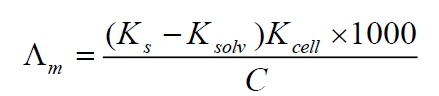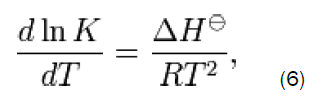All submissions of the EM system will be redirected to Online Manuscript Submission System. Authors are requested to submit articles directly to Online Manuscript Submission System of respective journal.

# Thermodynamics of Complex Formation ( conductometrically) Between Cu(II) ion and 4-phenyl -1- diacetyl monoxime 3 -thiosemicarbazone (BMPTS) in Ethanol at Different Temperatures

EA Gomaa*, KM Ibrahim, and NM Hassan.

Chemistry Department, Faculty of Science, Mansoura University, 35516 – Mansoura, Egypt

*Corresponding Author:
EA Gomaa
Chemistry Department, Faculty of Science
Mansoura University, 35516 – Mansoura, Egypt

Received date: 22/11/2013; Revised date: 15/12/2013; Published date: 29/12/2013

Visit for more related articles at Research & Reviews: Journal of Chemistry

## Abstract

The association constant ,formation constants and Gibbs free energies are calculated from the conductometric titration curves of CuCl2 with 4-phenyl-2-diacetyl monoxime-3-thiosemicarbazone (BMPTS) in absolute ethanol at different temperatures (293.15 K , 298.15 K , 303.15 and 308.15 K). On drawing the relation between molar conductance and the ratio of metal to ligand concentrations, different lines are obtained indicating the formation of 1:2 , 1:1 and 2:1 (M:L) stoichiometric complexes. The formation constants of different complexes in absolute ethanol follow the order: Kf (2:1) › Kf (1:1) >Kf (1:2) for (M: L). As the temperature increases, the formation constants and association constants of different complexes increase. The enthalpy and entropy of formation and association of CuCl2 with (BMPTS) were also estimated and their values were also discussed.

#### Keywords

Association constants; formation constants; Gibbs free energies; solvation free energies; enthalpy and entropy of solvation.

#### Introduction

Transition metal ions have a strong role in bio-inorganic chemistry and redox enzyme systems and may provide the basis of models for active sites of biological systems . Copper(II) ion is a biologically active, essential ion, cheating ability and positive redox potential allow participation in biological transport reactions. Cu(II) complexes possess a wide range of biological activity and are among the most potent antiviral, antitumor and anti inflammatory agents . The hydrazones and their metal complexes have many important applications in analytical chemistry and pharmacology [3-5]. Schiff bases hydrazone derivatives and their metal complexes have been studied for their interesting and important properties, e.g., antibacterial [6,7], antifungal , antioxidant , anticancer  and catalytic activity in oxidation of cyclohexene . Moreover, Schiff bases hydrazone derivatives are versatile ligands and they offer the possibility of different modes of coordination towards transition metal ions. Also, some of these derivatives have been applied as iron chelator drugs in therapy of anemia  and treatment of neuropathic pain [13-15].

This work deals with the Determination of solvation free energies (ΔG)s ,Enthalpy changes of solvation(ΔH)s and the entropy of solvation (ΔS) from conductometric technique. Thus, thermodynamic studies of complexation reactions of this Schiff base with transition metal ions is important on the thermodynamics of complexation reaction, but also lead to a better understanding of the high selectivity of this ligand towards different metal cations.

The aim of this work is the evaluation the non-covalent behavior of CuCl2 with (BMPTS) in absolute ethanol solutions at 294.15 K. These non-covalent interactions can help us for analysis of salts in bodies and environnement .

#### Experimental

Materials and Methods

All manipulations were performed under aerobic conditions. The cupper chloride and the used reagents were Merck pure.

Preparation of PDMSC

4-phenyl-1-diacetylmonoxime-3-thiosemicarbazone (BMPTS) was prepared by boiling an EtOH solution of 4- phenylthiosemicarbazone (1.7g, 1mmole) with an EtOH solution of diacetylmonoxime(1g, 1mmole) under reflux for two hours. The solution was then concentrated to one half of its volume and cooled. Yellowish white needles separated which were recrystallized from hot absolute EtOH. (M.p: 192 °C; yield 80%). The purity of the compound was checked by TLC.

Scheme 1: The outline of the synthesis of 4-phenyl-2-diacetyl monoxime-3-thiosemicarbazone (BMPTS)

Conductance measurement

The conductometric titration of the CuCl2 (1x10-4) mole/L against the ligand (1x10-3) mole/L in absolute ethanol was performed with 0.2 ml interval additions from (BMPTS) solution. The specific conductance values were recorded using conductivity bridge AdWa, AD 3000 with a cell constant equal to 1 cm-1. The temperature was adjusted at 293.15 K, 298.15 K, 303.15 K and 308.15 K.

#### Results and Discussion

The specific conductance values (Ks) of different concentrations of CuCl2 in absolute ethanol were measured experimentally in absence and in the presence of ligand at different temperatures (293.15 K , 298.15 K , 303.15 K and 308.15 K).

The molar conductance (Λm) values were calculated  using equation:Where Ks and Ksolv are the specific conductance of the solution and the solvent, respectively; Kcell is the cell constant and C is the molar concentration of the CuCl2 solutions.

The limiting molar conductances (ΛM) at infinite dilutions were estimated for CuCl2 in absolute ethanol alone at different temperatures by extrapolating the relation between Λm and Cm½ to zero concentration as shown in Figure 1.

Figure 1: The relation between molar conductance (Λm) and (Cm½) of CuCl2 alone in absolute ethanol at different temperatures (293.15K, 298.15 K, 303.15 K and 308.15 K).

The limiting molar conductances (Λo) at infinite dilutions were estimated for CuCl2 in the presence of the ligand (BMPTS) by extrapolating the relation between Λm and Cm½ to zero concentration Figure 2.

Figure 2: The relation between molar conductance (Λm) and (Cm½) of CuCl2 in presence of BMPTS at different temperatures (293.15K, 298.15 K, 303.15 K and 308.15 K).

By drawing the relation between molar conductance (/\m) and the molar ratio of metal to ligand (M/L) concentrations (Figure 3,4,5,6), different lines are obtained with sharp breaks indicating the formation of 1:2, 1:1 and 2:1 (M:L) stoichiometric complexes.

The experimental data of (ΛM) and (Λo) were analyzed for the determination of association and formation constants for each type of the stoichiometric complexes.

The association constants of CuCl2 in the presence of ligand (BMPTS) in absolute ethanol at different temperatures ( 293.15 K , 298.15 K , 303.15K and 308.15 K) for 2:1 ,1:1 and 1:2 (M:L) were calculated by using equation [17,18]:Where (Λm, Λ0) are the molar and limiting molar conductance of CuCl2 in presence of Hl respectively; Cm is molar concentration of CuCl2 , γ± is activity coefficient S(Z) is Fuoss-shedlovsky factor, equal with unity for strong electrolytes . The calculated association constants are shown in Table 1.

Table 1: Association constants of CuCl2 with (BMPTS) at different temperatures (293.15 K, 298.15 K, 303.15 K and 308.15 K).

The Gibbs free energies of association (ΔGA) were calculated from the association constant [20,21] by applying equation:

Δ GA = - R T ln KA (3)

Where R is the gas constant (8.341 J) and T is the absolute temperature .The calculated Gibbs free energies were presented in Table 2.

Table 2: Gibbs free energies of association of CuCl2 with (BMPTS) at different temperatures (293.15 K, 298.15 K, 303.15 K and 308.15 K).

The formation constants (Kf) for CuCl2 complexes were calculated for each type of complexes (1:2), (1:1) and (2:1) (M: L) [21,22] by using equation:Where ΛM is the limiting molar conductance of the CuCl2 alone, Λobs is the molar conductance of solution during titration and ΛML is the molar conductance of the complex.

The obtained values (Kf) for CuCl2-ligand stoichiometric complexes are presented in Table 3.

Table 3: Formation constants for 1:2, 1:1 and 2:1 (M/L) complexes in 80%V ethanol at different temperatures (293.15 K, 298.15 K, 303.15 K and 308.15 K).

The Gibbs free energies of formation for each stoichiometric complexes were calculated by using the equation:

ΔGf = - R T ln Kf (5)

The calculated ΔGf values are presented in Table 4.

Table 4: Gibbs free energies of formation of CuCl2 with (BMPTS) at different temperatures (293.15 K, 298.15 K, 303.15 K and 308.15 K).

The enthalpy (ΔHA) for CuCl2 complexes were calculated for each type of complexes (1:2) , (1:1) and (2:1) (M:L) by using van 't Hoff equation [23,24]:Where R is the gas constant (8.341 J) and T is the absolute temperature. By drawing the relation between log KA and 1/T, different lines are obtained indicating the formation of 1:2,1:1 and 2:1 (M:L) stoichiometric complexes Table 7.

Table 5: The enthalpies and entropies of association of complexes at different temperatures (293.15 K, 298.15 K, 303.15 K and 308.15 K).

Table 6: The enthalpies and entropies of formation of complexes at different temperatures (293.15 K, 298.15 K, 303.15 K and 308.15 K).

From the relation between log K and 1/T, ΔHA can be calculated for each type of complexes from the slope of each line (-ΔH/2.303R).The entropy (ΔSA) for CuCl2 complexes were calculated for each type of complexes (1:2), (1:1) and (2:1) (M:L) by using equation :

ΔG = ΔH – TΔS (7)

Where (S) is the entropy of system.

The calculated values of (ΔHA) and (ΔSA) for CuCl2-ligand stoichiometric complexes are presented in Table 5:

By drawing the relation between log Kf and 1/T, different lines are obtained indicating the formation of 1:2,1:1 and 2:1 (M:L) stoichiometric complexes Figure 8.

The enthalpy (ΔHf) for CuCl2 complexes were calculated for each type of complexes (1:2) , (1:1) and (2:1) (M:L) by using Van 't Hoff equation .

The calculated values of (ΔHf) and (ΔSf) for CuCl2-ligand stoichiometric complexes are presented in Table 6:

Since the conductance of an ion depends mainly on its mobility, it is quite reasonable to treat the rate process taking place with the change of temperature on the basis of equation(8):

where A is the frequency factor, R is the gas constant and Ea is the Arrhenius activation energy of the transfer process. Consequently, from the plot of logΛ0 vs. 1/T, the Ea values can be evaluated  as shown in Fig (9), giving high activation energy value due to solvation behavior.

The stability constants for the complexation of copper(II) ion with (BMPTS) were determined conductometrically at different temperatures. Thermodynamic parameters of complexation were determined from the temperature dependence of the formation constant. The negative values of ΔG show the ability of the studied ligand to form stable complexes and the process trend to proceed spontaneously. However, the obtained positive values of ΔH means that enthalpy is not the driving force for the formation of the complexes. Furthermore, the positive values of ΔS indicate that entropy is responsible for the complexing process. The formation constants and Gibbs free energies of different complexes follow that order: Kf (2:1) › Kf (1:1) › Kf (1:2) for (M:L), and ΔGf (2:1) › ΔGf (1:1) › ΔGf (1:2) for (M:L)